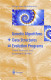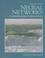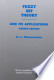### Fuzzy, Evolutionary and Neuro-computing

#### Course Description

Fuzzy, evolutionary and neuro-computation is a group of methods that differ from classical computational methods in very fundamental ideas. They are based on approximate reasoning, self learning, parallelism and non-determinism. Inspiration for these methods comes from biology e.g. biological neuron, process of evolution, human like approximate reasoning etc. These methods can solve problems that cannot be solved by applying classical mathematical and computational methods and they are used in scientific research and in myriad applications and everyday products.

#### General Competencies

Students will acquire theoretical foundations, they will recognise possible sutability of the presented methods for the particular problem and will obtain skills in appling fuzzy, evolutionary and neurocomputing methods in variety of scientific, control, financial problems.

#### Learning Outcomes

1. describe and define basic areas of soft computing
2. apply fuzzy logic models on control problems
3. apply artificial neural networks for prediction and classification tasks
4. solve optimization problems using evolutionary computation
5. combine different soft computing techniques into complete system
6. select the appropriate soft computing method for solving various problems

#### Forms of Teaching

Lectures

There are 15 weeks for lectures (2 hours each). Two of these 15 weeks are used for exams. Lectures are divided in two cycles. After each cycle there is an exam.

Exams

There is one midterm exam and one final exam.

Seminars

Student will have to finish one practical assignment which will be demonstrated to lecturer.

Continuous Assessment Exam
Seminar/Project 0 % 20 % 0 % 0 %
Mid Term Exam: Written 0 % 40 % 0 %
Final Exam: Written 0 % 40 %
Exam: Written 50 % 50 %
Exam: Oral 50 %

#### Week by Week Schedule

1. Introduction to fuzzy, evolutionary and neuro-computation. History of the field. Biologicaly inspired computation. Applications and research topics.
2. Fuzzy sets, standard notation, properties, examples. Standard min-max operations, S and T norms. Fuzzy relations.
3. Fuzzy relations composition, fuzzy graphs. Linguistic variables, linguistic hedges, fuzzy if-then rules.
4. Extension principle. Interval and fuzzy aritmetics. Metrics.
5. Fuzzy logic, fuzzy rules, generalised modus ponens, extension of fuzzy logic - fuzzy reasoning.
6. Fuzzy control systems, fuzzy system structure, types of rules and reasoning, fuzzification and defuzzification methods. case studies.
7. Introduction to neural networks. Types of networks and learning methods. Feedforward neural network and backpropagation algorithm.
8. Midterm exam
9. Self-organizing neural networks and training algorithms.
10. Combining neural network and fuzzy logic: neuro-fuzzy systems.
11. Genetic algorithms and evolution programs. Principle of evolution. Genetic code. Representation. Initial population. Evaluation function. Genetic operators: selection, crossover and mutation. Genetic algorithm parameters.
12. Simple GA. Schema theorem and building block hypothesis. Genetic algorithm in practise. Numerical optimisation.
14. Using GA for fuzzy model identification. Course summary.
15. Final exam.

#### Study Programmes

Computer Engineering (profile)
Recommended elective courses (3. semester)
Computer Science (profile)
Specialization Course (1. semester) (3. semester)
Software Engineering and Information Systems (profile)
Recommended elective courses (3. semester)
Telecommunication and Informatics (profile)
Recommended elective courses (3. semester)

#### LiteratureZ.Michalewicz (1996.), Genetic Algorithms + Data Structures = Evolution Programs, Springer Verlag, Berlin, 3rd ed.S.Haykin (1999.), Neural Networks, Comprehensive Foundation, Prentice Hall, 2nd ed.
J. Yen and R. Langari (1999.), Fuzzy Logic, Prentice HallH.J.Zimmermann (2001.), Fuzzy Set Theory and Its Applications, Kluwer Academic Publishers, 4th ed.
M. Čupić. B. Dalbelo Bašić, M. Golub (2012.), Neizrazito, evolucijsko i neuroračunarstvo,
(.), Handbook of Genetic Algorithms;L. Davis;1991;Van Nostrand Reinhold, New York,

#### General

ID 222767
Winter semester
4 ECTS
L0 English Level
L1 e-Learning
30 Lectures
13 Laboratory exercises